Your data analysis solution

# Latent Class Regression Model in Excel tutorial

2016-05-17

This tutorial will show you how to run a Latent Class regression model in Excel using the XLSTAT statistical software.

## What are latent class regression models?

This tutorial shows how to develop Latent Class Regression models. You will learn how to:

• Select the dependent variable and specify its scale type
• Determine the number of latent classes (i.e., segments)
• Explore the Parameters output

In addition, this example illustrates several advanced options in the XLSTAT-LatentClass Latent Class Regression tool. You will learn how to:

• Use the optional case ID variable to specify repeated observations
• Classify cases into latent segments

## Dataset for estimating latent class regression models

An Excel sheet containing the data for use in this tutorial can be downloaded by clicking here.

The data for this example are obtained from a hypothetical conjoint marketing study involving repeated measures where respondents were asked to provide likelihood of purchase ratings under each of several different scenarios. A partial listing of the data is shown in Figure 1.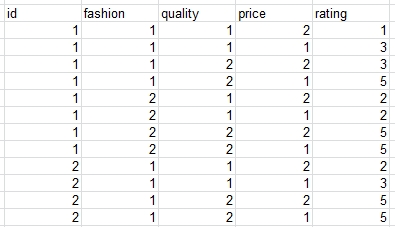Figure 1:  Partial Listing of Conjoint Data

As suggested in Figure 1, there are 8 records for each case (there are 400 cases in total); one record for each cell in this 2x2x2 complete factorial design of different scenarios for the purchase of a product:

• FASHION (1 = Traditional; 2 = Modern)
• QUALITY (1 = Low; 2 = High)
• PRICE (1 = Lower; 2 = Higher)

The dependent variable (RATING) is a rating of purchase intent on a five-point scale. The three attributes listed above will be used as predictor variables in the model.

## Goal of this tutorial on Latent Class regression models

Use XLSTAT-LatentClass latent class regression to identify latent segments differing with respect to the estimate of importance attached to each of the three attributes, which influence an individual’s purchase decision. The Latent Class regression model allows for the fact that these estimates may differ for different segments. That is, for one segment, price and only price may influence the decision, while a second segment may be influenced by quality and modern appearance, but is price insensitive. We will treat RATING as an ordinal dependent variable and compare several models to determine the number of segments.

## Setting up Latent Class regression Models in XLSTAT-LatentClass

To activate the XLSTAT-LatentClass regression dialog box, select the XLSTAT / XLSTAT-LatentClass / Latent class regression command in the Excel menu (see Figure 2).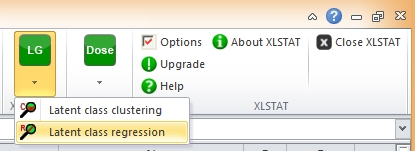Figure 2: Opening XLSTAT-LatentClass Regression

Once you have clicked the button, the XLSTAT-LatentClass regression dialog box is displayed.

The Latent Class Regression Analysis dialog box, which contains 5 tabs, opens (see Figure 3).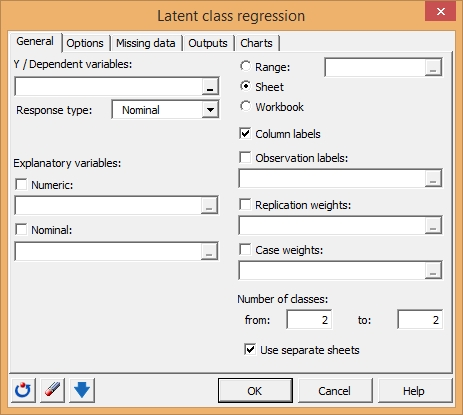Figure 3: Analysis Dialog Box for LC Regression Model

For this analysis, RATING will be the dependent variable.

In the Y / Dependent Variable field, select the variable RATING.

We also need to indicate the dependent variable scale type. For this example, we will use the scale type (Ordinal-Fixed) which takes into account the natural ordering between the 5 levels of purchase intent. By default, the fixed scores on the data (1, 2, 3, 4 and 5) are used which order the levels and establish equal distance between adjacent levels.

From the Response Type drop down menu, select ‘Ordinal’.

As explained above, the data contains repeated observations for each respondent (case). Therefore, we need to indicate which records belong to each case. This is accomplished using a Case ID variable, which contains a unique identification number for each case. All records belonging to the same case are assigned the same unique ID.

Check the box for ‘Observation labels’ and then in the corresponding field, select the variable ID.

Next, we will select the Predictors. Predictors are used as independent variables in the regression model. In the current example, we use the product attributes FASHION, QUALITY and PRICE as predictors.

Check the box for ‘Nominal’ and then in the corresponding field, select the variables 'FASHION', 'QUALITY' and 'PRICE'.

The Latent Class regression model simultaneously estimates a separate regression model for each class. A 1-class model estimates only a single regression model. It makes the standard homogeneity assumption that a single regression model holds true for all cases. In the current example, we will start by estimating a 1-class model and obtain a log-likelihood statistic to be used as a base. We will then estimate additional models, which successively increment the number of classes by 1 and assess the significance of each additional class.

One assessment consists of a check of whether the change in the log-likelihood for each pair of successive models fails to decrease by a significant amount as determined by the BIC statistic. (The model having the lowest BIC might then be selected.) A second assessment is to utilize the p-value associated with the L2 fit statistic.

Request the estimation of 4 different LC Regression models – a 1-class model, a 2-class model, a 3-class model and a 4-class model:

Under Number of Classes, in the box titled ‘from:’ type ‘1’ and in the box titled ‘to’ type ‘4’.

Your Analysis Dialog Box should now look like this: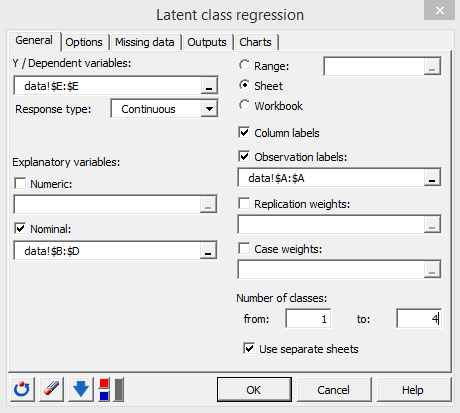Figure 4: Regression Analysis Dialog Box with Initial Settings

The fast computations start when you click on OK.

## Interpreting a Latent Class regression model output in XLSTAT-LatentClass

When XLSTAT-LatentClass completes the estimation, 5 spreadsheets will be produced – a Regression Summary  sheet (Latent class regression), and a sheet for each of the latent class models estimated (1-class model (LCR-1 Class), a 2-class model (LCR-2 Classes), a 3-class model (LCR-3 Classes) and a 4-class model (LCR-4 Classes)).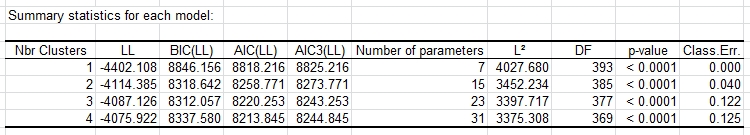Figure 5: Summary of Models Estimated

This output reports statistics that will assist you in determining the correct number of classes -- the loglikelihood (LL) values, the BIC values, and the number of parameters in the estimated models. It is important to determine the right number of classes because specifying too few ignores class differences, while specifying too many may cause the model to be unstable. While the log-likelihood increases each time the number of classes is increased, the minimum BIC value occurs for Model3 (BIC=8312.057), suggesting that the 3-class solution is the best of the four estimated models.

Occasionally, you might obtain a local (suboptimal) solution. For these data, it is possible to obtain a local solution for the 4-class model, obtaining LL = -4080.318 instead of –4075.922. If this occurs, re-estimate the 4-class model.

Note: Notice that the p-values based on the model L2 and reported degrees of freedom (df) are not valid assessments of fit because we are dealing with sparse data.

We will now examine the detailed output for the 3-class solution.

Click on the sheet ‘LCR-3 Classes’ to view the model output for the 3-class model.

Following the summary statistics output section, the output is presented.

## Parameters Output

First, we will view the Parameters output

scroll down to the Parameters output (see Figure 6).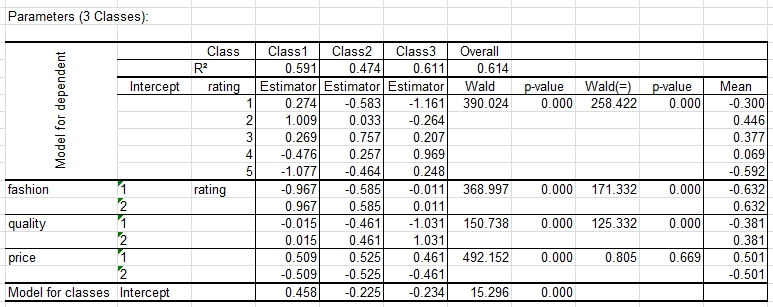Figure 6: Parameters Output for 3-Class Model

The beta parameter for each predictor is a measure of the influence of that predictor on RATING. The beta effect estimates under the column labeled ‘Class 1’ suggest that segment 1 is influenced in a positive way by products for which FASHION = 2 (beta = 0.967), in a negative way by a higher PRICE (beta = -0.509), and not at all by higher QUALITY (beta is approximately 0). We also see that segment 2 (‘Class 2’) is influenced by all 3 attributes, having a preference for those product choices that are modern (beta = 0.585), and higher quality (beta = 0.461), but, like segment 1, their preference also decreases as a function of price (beta = -0.525). Members of segment 3 prefer higher quality products (beta = 1.031), but their preference also decreases as a function of price (beta = -0.461), and they are not influenced by FASHION.

Note that PRICE has more or less the same influence on all three segments. The Wald(=) statistic indicates that the differences in these beta effects across classes are not significant (the p-value = .67 which is much higher than .05, the standard level for assessing statistical significance). This means that all 3 segments exhibit price sensitivity to the same degree. This is confirmed when we estimate a model in which this predictor is specified to be class-independent (check the corresponding option under the Options tab). The p-value for the Wald statistic for PRICE is .000 to 3 decimal places. Clicking on this value we see that the p-value more precisely is 2.4x10-106 indicating that the amount of price sensitivity is highly significant.

With respect to the effect of the other two attributes we find large between-segment differences. The predictor FASHION has a strong influence on segment 1, a less strong effect on segment 2, and virtually no effect on segment 3. QUALITY has a strong effect on segment 3, a less strong effect on segment 2, and virtually no effect on segment 1. The fact that the influence of FASHION and QUALITY differs significantly between the 3 segments is confirmed by the significant p-values associated with the Wald(=) statistics for these attributes. For example, for FASHION, the p-value = 6.2x10-38.

In summary, segment 1 could be labeled the “Fashion-Oriented Segment”, segment 3 the “Quality-Oriented Segment”, and segment 2 is the segment that takes into account all 3 attributes in their purchase decision.

Copyright ©2015 Statistical Innovations Inc. All rights reserved.

### Contact Us

#### Contact our technical support team: support@xlstat.com

https://cdn.desk.com/
false
desk
Loading
seconds ago
a minute ago
minutes ago
an hour ago
hours ago
a day ago
days ago
about
false
Invalid characters found
/customer/portal/articles/autocomplete
9283